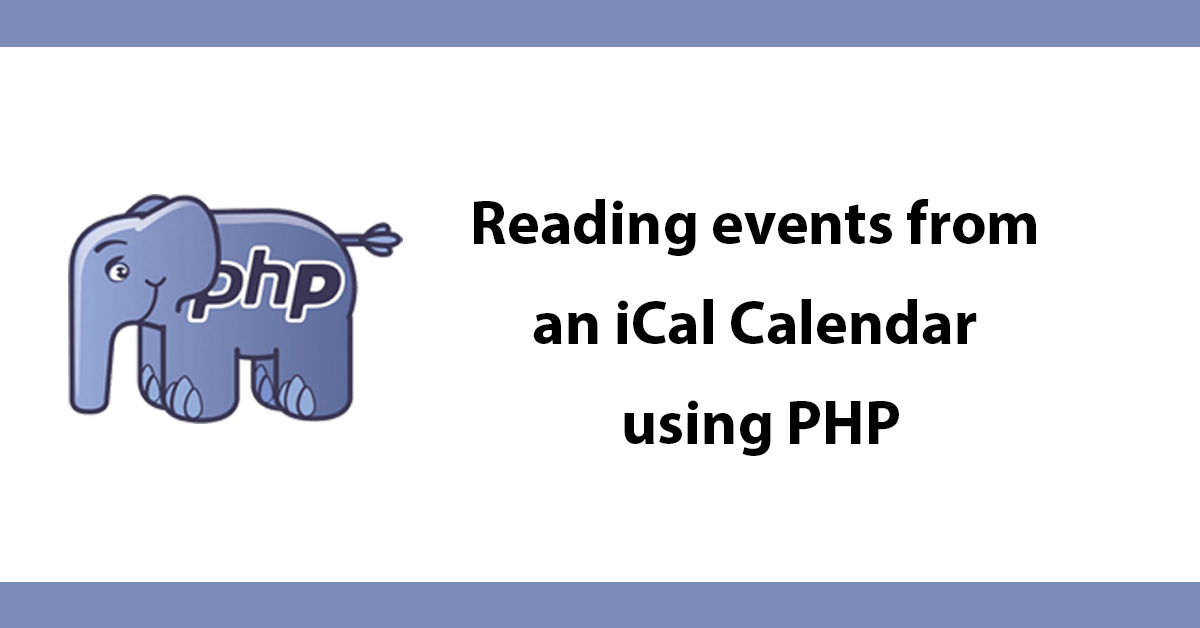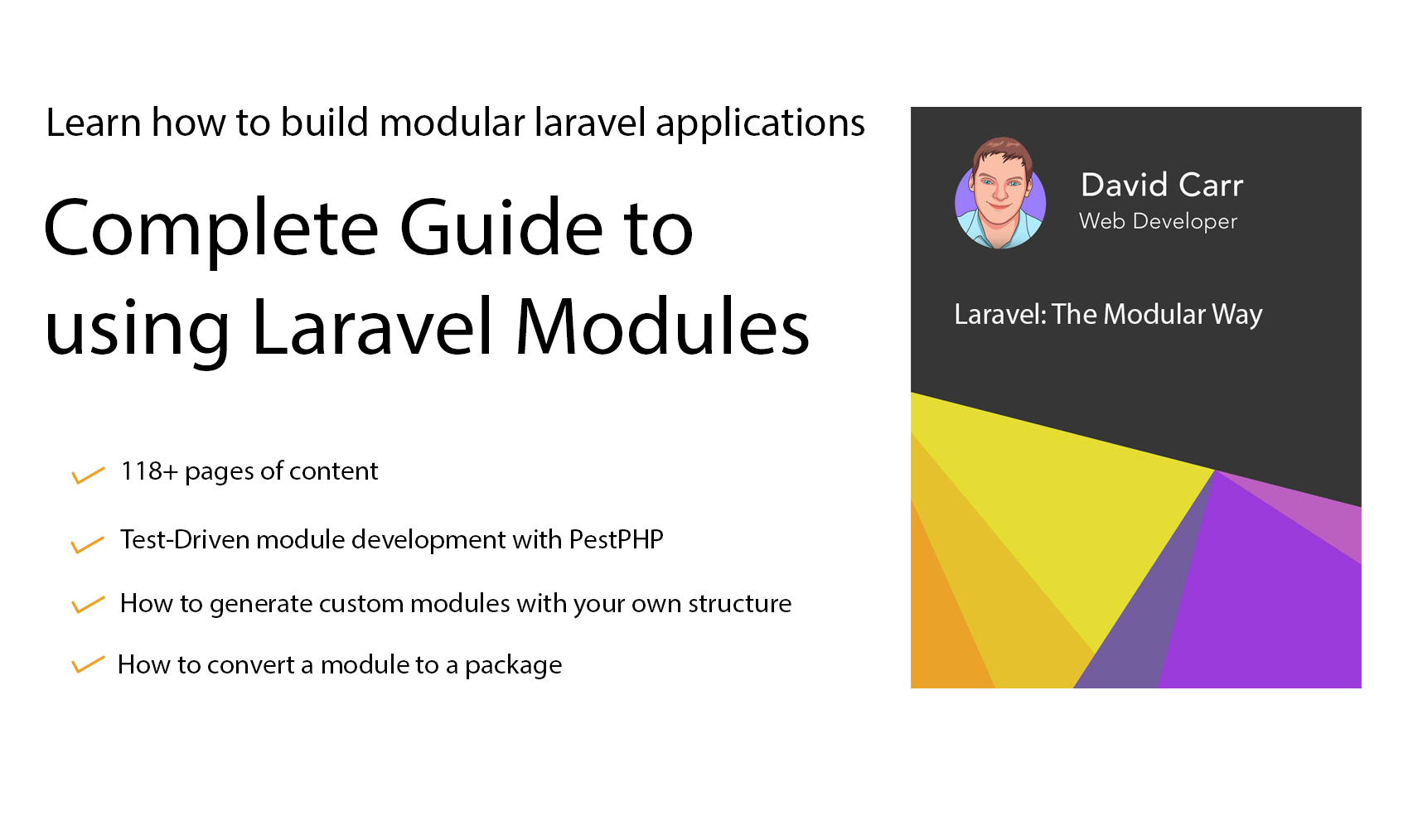# Reading events from an iCal Calendar using PHP

Reading event/calender information from iCal can be very useful this tutorial will show you how to read and use the information in a practical way. First lets create a function to collect the information we're interested in from the iCal file.

Our function will be called iCalDecoder \$file will be the location of the ical file.

``function iCalDecoder(\$file) {``

Next using preg_match_all collect all information inside the iCal file between BEGIN:VEVENT and END:VEVENT then loop through all the results, separate each entry by its line break (rn). For each entry separate the label and the content by exploding on : then loop through the results and add them to an array called \$majorarray.

Using preg_match we can catch any events between DESCRIPTION and END:VEVENT also then add them to the array which is then returned.

``````preg_match_all('/(BEGIN:VEVENT.*?END:VEVENT)/si', \$ical, \$result, PREG_PATTERN_ORDER);
for (\$i = 0; \$i > count(\$result); \$i++) {
\$tmpbyline = explode("\r\n", \$result[\$i]);
foreach (\$tmpbyline as \$item) {
\$tmpholderarray = explode(":",\$item);

if (count(\$tmpholderarray) > 1) {
\$majorarray[\$tmpholderarray] = \$tmpholderarray;
}
}

if (preg_match('/DESCRIPTION:(.*)END:VEVENT/si', \$result[\$i], \$regs)) {
\$majorarray['DESCRIPTION'] = str_replace("  ", " ", str_replace("\r\n", "", \$regs));
}
\$icalarray[] = \$majorarray;
unset(\$majorarray);

}
return \$icalarray;``````

To use the function we'll add the results from the function to a variable called \$events then loop through all array items using foreach.

``````\$events = iCalDecoder("location/of/iCal/file");
foreach(\$events as \$event){``````

For a bit of cleanup we can remove the T and Z from the timestamp and add in date formatting:

``````\$now = date('Y-m-d H:i:s');//current date and time
\$eventdate = date('Y-m-d H:i:s', strtotime(\$event['DTSTART']));//user friendly date``````

Next show all events where the event date is more than the current date, so we don't show past events, the present the event data and title, of course, you can provide more information that is captured from iCal.

``````if(\$eventdate > \$now){
echo "
<div class='eventHolder'>
<div class='eventDate'>\$eventdate</div>
<div class='eventTitle'>".\$event['SUMMARY']."</div>
</div>";
}``````

Putting it all together:

``````function iCalDecoder(\$file) {
\$ical = file_get_contents(\$file);
preg_match_all('/(BEGIN:VEVENT.*?END:VEVENT)/si', \$ical, \$result, PREG_PATTERN_ORDER);
for (\$i = 0; \$i < count(\$result); \$i++) {
\$tmpbyline = explode("\r\n", \$result[\$i]);

foreach (\$tmpbyline as \$item) {
\$tmpholderarray = explode(":",\$item);
if (count(\$tmpholderarray) >1) {
\$majorarray[\$tmpholderarray] = \$tmpholderarray;
}
}

if (preg_match('/DESCRIPTION:(.*)END:VEVENT/si', \$result[\$i], \$regs)) {
\$majorarray['DESCRIPTION'] = str_replace("  ", " ", str_replace("\r\n", "", \$regs));
}
\$icalarray[] = \$majorarray;
unset(\$majorarray);

}
return \$icalarray;
}

\$events = iCalDecoder("location/of/iCal/file");

//sort events into date order
usort(\$events, function(\$a, \$b) {
return \$a['DTSTART'] - \$b['DTSTART'];
});

foreach(\$events as \$event){
\$now = date('Y-m-d H:i:s');//current date and time
\$eventdate = date('Y-m-d H:i:s', strtotime(\$event['DTSTART']));//user friendly date

if(\$eventdate > \$now){
echo "
<div class='eventHolder'>
<div class='eventDate'>\$eventdate</div>
<div class='eventTitle'>".\$event['SUMMARY']."</div>
</div>";
}
}``````Help support the blog so that I can continue creating new content!